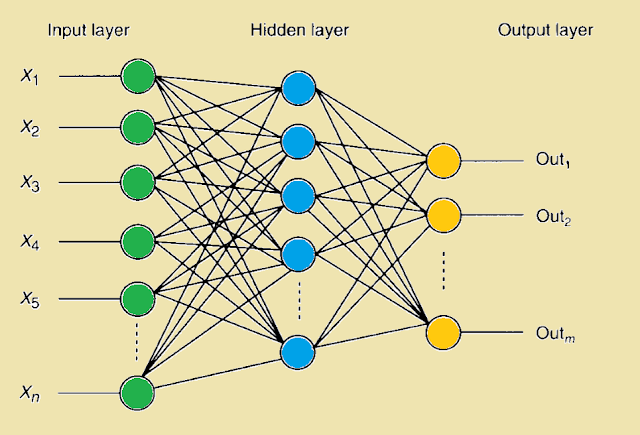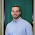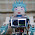## 2017年3月26日 星期日

### 深度學習(2)--使用Tensorflow實作卷積神經網路(Convolutional neural network，CNN)

卷積神經網路(Convolutional neural network，CNN)，是一多層的神經網路架構，是以類神經網路實現的深度學習，在許多實際應用上取得優異的成績，尤其在影像物件識別的領域上表現優異。

傳統的多層感知器(Multilayer Perceptron，MLP)可以成功的用來做影像識別，如之前

例如，考慮MNIST的手寫輸入資料為28x28解析度，考慮神經元全連接的方式
(Full connectivity)，連接到第一個隱藏層神經元便需要28x28=784個權重(Weight)。如果考慮一
RGB三通道色彩圖像輸入，如CIFAR-10為32x32x3的圖像解析度，連接到第一個隱藏層神經

像這樣子的網路架構，沒有考慮到原始影像資料各像素(pixel)之間的遠近，或是密集關係，只是一昧的將全部像素(pixel)當各個輸入的特徵值，連接到隱含層神經元，造成爆量增加的權重不僅是一種浪費，因為實際上不須這麼多的權重，效果不僅沒增加，只是增加了運算的負荷，而且很可能會造成過度適合(Overfitting)。而卷積神經網路(Convolutional neural network，
CNN)被提出來後，可以有效的解決此一問題。

事實上卷積神經網路(Convolutional neural network，CNN)設計的目標就是用來處理以多陣列型態表達的資料，如以RGB三通道表達的彩色圖片。CNN和普通神經網路之間的一個實質差別在於，CNN是對原始圖像直接做操作，而傳統神經網路是人為的先對影像提取特徵(例如灰階化，二值化)才做操作。

CNN有三個主要的特點。

1.感知區域(Receptive field)：可採用3維的圖像資料(width，height，depth)與神經元連接方式，
實際上也可以直接採用2維的圖像資料，但隱含層內部的神經元只與原本圖像的某一小塊
區域做連結。該區塊我們稱之為感知區域(Receptive field)

2.局部連結採樣(Local connectivity)：根據上述感知區域(Receptive field)的概念，CNN使用過濾
器(filters)增強與該局部圖形空間的相關性，然後堆疊許多這樣子的層，可以達到非線性
濾波的功能，且擴及全域，也就是允許網路架構從原圖小區塊的較好的特徵值代表性，組
合後變成大區塊的特徵值代表性。

3.共享權重(Shared weights)：使用的過濾器(filters)是可以重複使用的，當在原始圖像產生一
特徵圖(Feature Map)時，其權重向量(weights vector)及偏誤(bias)是共用的。這樣可以確保
在該卷積層所使用的神經元會偵測相同的特徵。並且即使圖像位置或是有旋轉的狀態，
仍然可以被偵測。

這三個特點使得CNN在圖像辨識上有更好的效果。在實際操作上的三個基礎分別是：
區域感知域(Local Receptive field)，卷積(Convolutional )，池化(pooling)。
我們可以從下面圖形化的實際操作來理解它的意思。

下圖1，2說明在卷積層的運作方式，假設原始影像為一32x32x3維度，我們可以任意給定一

接著與原圖像相同的小區域計算點積，得到的一個值便是在新的feature Map的一個新元素值。卷積核會在原始的圖像或者前一個圖像(Map)按照Stride(步伐)的設定移動，直到掃描完全部圖像區域，這樣便會產生一個新的Feature Map。便是下圖圖3~圖5所示，在這個步驟中產生單一新feature Map所使用的權重W，也就是卷積核是共用的，並且共用同一個偏誤(bias)，通常初始預設偏誤(bias)可以為0。這樣確保這一新的feature map偵測的是該原始圖像同一特徵。

<圖1>

<圖2>
<圖3>
<圖4>
<圖5>

這邊要注意，上圖3~5只是單純說明當卷積操作時如何作點積取和，實際在CNN神經網路運算裡，產生的新Feature Map裡的單一元素便是連接到一個神經元做運算，故其運算應當如下公式，這就是一般神經網路向前傳遞的公式形式：

W便是kxk維卷積核，Xij為前一個圖像的元素值，i,j為其index。
b為偏誤(bias) 預設可以為0。
S()為一激勵函數可以為Sgmoid或ReLu或其他函數，如下圖7。
y為輸出的值，便是產生一新feature Map上其中的一個元素值。

另外要注意的是，卷積層要產生幾個新的feature map個數是在網路架構初始化指定的，而要產生幾個新的feature Map，便要使用相同數量的不同卷積核。例如下圖8及圖9所示，使用
6個不同的卷積核便可產生6個feature Map。

而feature map的大小是由卷積核和上一層輸入圖像的大小決定的，假設上一層的圖像大小是n*n、卷積核的大小是k*k，則該層的feature map大小是(n-k+1)*(n-k+1)，比如上圖3~5，從7*7的圖像大小，產生3x3 feature map  (3=7-5+1），當然這是在預設stride=1的情狀下。

<圖6>Stride=1，卷積後產生一個Activation Map，也就是Feature Map。

<圖7>常見激勵函數<圖8>使用第二個不同的卷積核(Filter)產生第二個feature map

至此，我們來以下圖9計算一下使用了多少參數(w權重及b偏誤)，及多少連接到神經元。

6個新feature map所使用參數數量為：6x(5x5+1)=156個參數

有關卷積(Convolutional)的操作也可以參閱底下一般影像處理領域的操作。其操作本質上便

OPENCV(7)--2D Convolution ，Image Filtering and Blurring (旋積，濾波與模糊)

<圖9>使用6個不同的卷積核(Filter)，產生6個feature map

底下圖10是用來說明不同的Stride設定，在卷積後，所產生的新Map尺寸便會不同。

<圖10>

<圖11>

池化層緊接在卷積層之後，是將前一層的輸入資訊作壓縮。池化通常使用2x2核並且stride=2

Max pooling的操作方式。另外通常在這一層不再作計算權重及偏誤以及激勵函數。只單純Max pooling。

<圖12>
<圖13>

CNN神經網路架構，最後仍有全連接層(Fully connected Layer)，其操作其運算方式便如同一般的MLP方式。整個CNN神經網路仍然使用BP反向傳遞演算法計算誤差後更新權重。

<圖14>全連接層def conv2d(img, w, b):
(tf.nn.conv2d(img, w,\
strides=[1, 1, 1, 1],\

<圖15>自訂一個CNN網路架構

# Parameters
learning_rate = 0.001
training_iters = 400000 #迭帶400000次
batch_size = 128   #使用minibatch方式
display_step = 10

# Network Parameters
n_input = 784 # MNIST data input (img shape: 28*28)
#原本MNIST數據集為1x784，會被reshape回28x28，當作原始輸入
n_classes = 10 # MNIST total classes (0-9 digits)
dropout = 0.75 # Dropout, probability to keep units

#dropout = 0.75意義為：為了減少過度適合(Overfitting)的問題，應用了丟棄(dropout)正則化技術。
#意旨在神經網路中丟棄一些連接的單元(輸入，隱藏，和輸出)，決定丟棄那些神經元是隨機
#的也可以用機率決定。

wc1 = tf.Variable(tf.random_normal([5, 5, 1, 32])) # 5x5 conv, 1 input, 32 outputs
#32代表第1個卷積層要產生32個新的feature map
wc2 = tf.Variable(tf.random_normal([5, 5, 32, 64])) # 5x5 conv, 32 inputs, 64 outputs
#64代表第2個卷積層要產生64個新的feature map
#5,5則是5x5的卷積核(filter權重)
wd1 = tf.Variable(tf.random_normal([7*7*64, 1024])) # fully connected, 7*7*64 inputs, 1024 outputs
wout = tf.Variable(tf.random_normal([1024, n_classes])) # 1024 inputs, 10 outputs (class prediction)
#1024則是全連接層的1024個神經元數量，n_classes=10，代表0-9的數字類別

# Convolution Layer
conv1 = conv2d(_X,wc1,bc1)
conv1 = max_pool(conv1, k=2)
conv1 = tf.nn.dropout(conv1,keep_prob)
#定義第1層卷積層及pooling層並使用dropout正則化

# Convolution Layer
conv2 = conv2d(conv1,wc2,bc2)
conv2 = max_pool(conv2, k=2)
conv2 = tf.nn.dropout(conv2, keep_prob)
#定義第2層卷積層及pooling層並使用dropout正則化

# Fully connected layer
dense1 = tf.reshape(conv2, [-1, wd1.get_shape().as_list()]) # Reshape conv2 output to fit dense layer input
dense1 = tf.nn.relu(tf.add(tf.matmul(dense1, wd1),bd1)) # Relu activation
dense1 = tf.nn.dropout(dense1, keep_prob) # Apply Dropout
#定義F5層全連接層使用ReLu激勵函數及dropout正則化

cost = tf.reduce_mean(tf.nn.softmax_cross_entropy_with_logits(labels=y, logits=pred))
#計算成本函數的方式並使用softmax_cross_entropy_with_logits
#注意，在Tensorflow R1.0.1版，注意這兩個參數(labels=y, logits=pred)的放法跟以前版本不同
<圖16>底下為訓練結果及測試結果

<完整程式>
```# Import MINST data
import input_data
import tensorflow as tf

# Parameters
learning_rate = 0.001
training_iters = 400000
batch_size = 128
display_step = 10

# Network Parameters
n_input = 784 # MNIST data input (img shape: 28*28)
n_classes = 10 # MNIST total classes (0-9 digits)
dropout = 0.75 # Dropout, probability to keep units

# tf Graph input
x = tf.placeholder(tf.float32, [None, n_input])
y = tf.placeholder(tf.float32, [None, n_classes])
keep_prob = tf.placeholder(tf.float32) #dropout (keep probability)

# Create model
def conv2d(img, w, b):
(tf.nn.conv2d(img, w,\
strides=[1, 1, 1, 1],\

def max_pool(img, k):
return tf.nn.max_pool(img, \
ksize=[1, k, k, 1],\
strides=[1, k, k, 1],\

# Store layers weight & bias

wc1 = tf.Variable(tf.random_normal([5, 5, 1, 32])) # 5x5 conv, 1 input, 32 outputs
wc2 = tf.Variable(tf.random_normal([5, 5, 32, 64])) # 5x5 conv, 32 inputs, 64 outputs
wd1 = tf.Variable(tf.random_normal([7*7*64, 1024])) # fully connected, 7*7*64 inputs, 1024 outputs
wout = tf.Variable(tf.random_normal([1024, n_classes])) # 1024 inputs, 10 outputs (class prediction)

bc1 = tf.Variable(tf.random_normal())
bc2 = tf.Variable(tf.random_normal())
bd1 = tf.Variable(tf.random_normal())
bout = tf.Variable(tf.random_normal([n_classes]))

# Construct model
_X = tf.reshape(x, shape=[-1, 28, 28, 1])

# Convolution Layer
conv1 = conv2d(_X,wc1,bc1)
# Max Pooling (down-sampling)
conv1 = max_pool(conv1, k=2)
# Apply Dropout
conv1 = tf.nn.dropout(conv1,keep_prob)

# Convolution Layer
conv2 = conv2d(conv1,wc2,bc2)
# Max Pooling (down-sampling)
conv2 = max_pool(conv2, k=2)
# Apply Dropout
conv2 = tf.nn.dropout(conv2, keep_prob)

# Fully connected layer
dense1 = tf.reshape(conv2, [-1, wd1.get_shape().as_list()]) # Reshape conv2 output to fit dense layer input
dense1 = tf.nn.relu(tf.add(tf.matmul(dense1, wd1),bd1)) # Relu activation
dense1 = tf.nn.dropout(dense1, keep_prob) # Apply Dropout

# Output, class prediction

#pred = conv_net(x, weights, biases, keep_prob)
# Define loss and optimizer
cost = tf.reduce_mean(tf.nn.softmax_cross_entropy_with_logits(labels=y, logits=pred))

# Evaluate model
correct_pred = tf.equal(tf.argmax(pred,1), tf.argmax(y,1))
accuracy = tf.reduce_mean(tf.cast(correct_pred, tf.float32))

# Initializing the variables
#init = tf.initialize_all_variables()
init =tf.global_variables_initializer()
# Launch the graph
with tf.Session() as sess:
sess.run(init)
step = 1
# Keep training until reach max iterations
while step * batch_size < training_iters:
batch_xs, batch_ys = mnist.train.next_batch(batch_size)
# Fit training using batch data
sess.run(optimizer, feed_dict={x: batch_xs, y: batch_ys, keep_prob: dropout})
if step % display_step == 0:
# Calculate batch accuracy
acc = sess.run(accuracy, feed_dict={x: batch_xs, y: batch_ys, keep_prob: 1.})
# Calculate batch loss
loss = sess.run(cost, feed_dict={x: batch_xs, y: batch_ys, keep_prob: 1.})
print ("Iter " + str(step*batch_size) + ", Minibatch Loss= " + "{:.6f}".format(loss) + ", Training Accuracy= " + "{:.5f}".format(acc))
step += 1
print ("Optimization Finished!")
# Calculate accuracy for 256 mnist test images
print ("Testing Accuracy:", sess.run(accuracy, feed_dict={x: mnist.test.images[:1024], y: mnist.test.labels[:1024], keep_prob: 1.}))

```

<參考資料>
書名：深度學習快速入門，作者：Giancarlo Zaccone 傅運文譯

<其他相關文章>

#### 6 則留言:

1.Hello Ashing, thank you for the nice blog posts. Very clear and well presented. Are you based in Hong Kong?

1.I am from Taiwan,thanks.

2.感謝, 這篇文章讓我更清楚 CNN 在影像辨識方面應用和架構

3.CNN和普通神經網路之間的一個實質差別在於，CNN是對原始圖像直接做操作，而傳統神經網路是人為的先對影像提取特徵(例如灰階化，二值化)才做操作。 -- 這是不是寫反了?

4.請問 Ashing 蔡兄, 應用CNN到圖形辨識上 您講的精彩
那麼如何應用CNN到語音辨識呢?

福祥 fsptsen@gmail.com

1.基本上CNN是圖形辨識,語音辨識需要用RNN,兩者用途有些區隔!